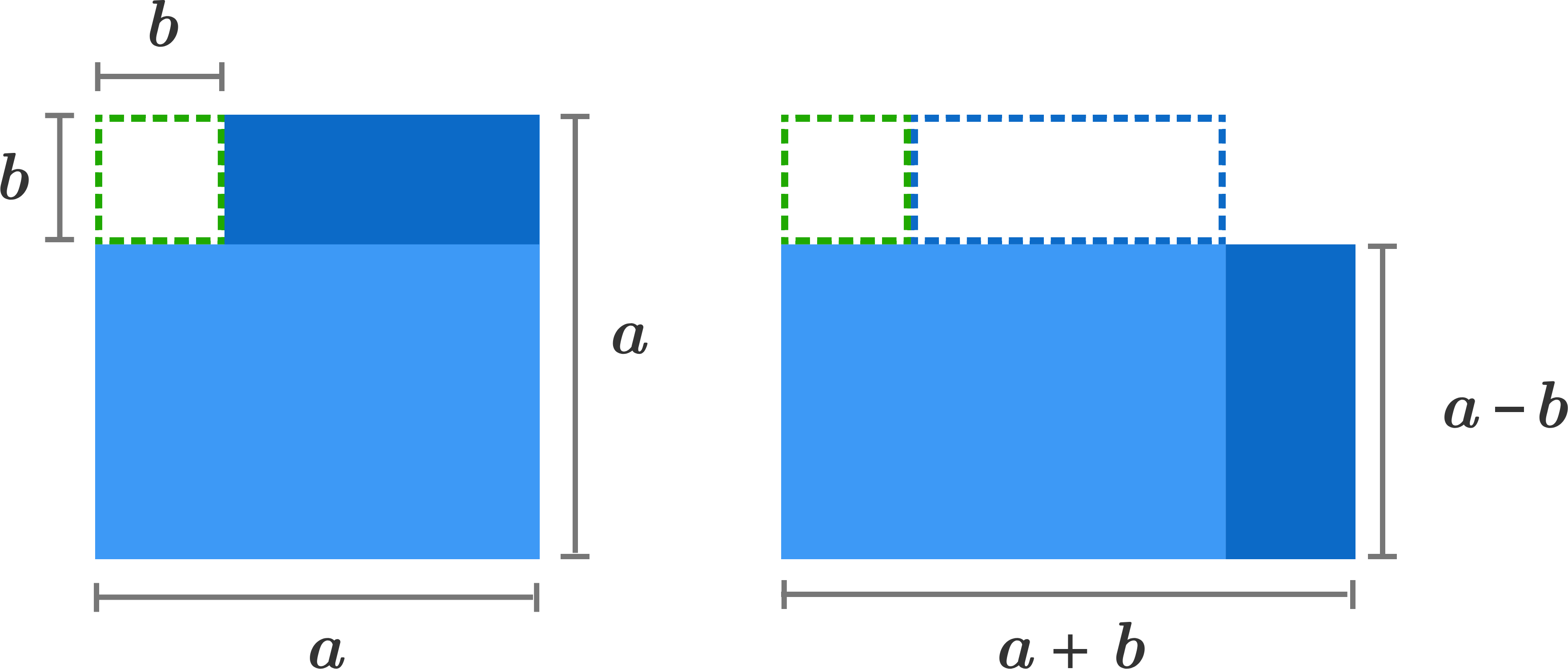Algebra I

Here are some situations where quick shortcuts can save you a lot of time and help you avoid annoying arithmetic.

Simplify $\large \frac{ {\color{#20A900}{2}} ^ {\color{#3D99F6}{3}} \times {\color{#20A900}{2}} ^ {\color{#3D99F6}{7}}}{ {\color{#20A900}{2}} ^ {\color{#3D99F6}{6}} \times {\color{#20A900}{2}} ^ {\color{#3D99F6}{3}} }.$

Note: You are not a robot, and you don't need a calculator to solve this!Exponent Shortcuts and Misconceptions

Which is larger,

$\large 3 ^ { 4 } + 3 ^ { 4 } + 3 ^ { 4 } \hspace{.5cm} \text{ or } \hspace{.55cm} {3} ^ { 5} \, ?$

Exponent Shortcuts and Misconceptions

Evaluate

$\large \frac{ \color{#3D99F6}{42} ^ 2 - \color{#20A900}{38} ^ 2 } { \color{#3D99F6}{42} - \color{#20A900}{38} }.$

Hint: Use the difference of two squares identity: $a^2-b^2=(a+b)(a-b)$.Exponent Shortcuts and Misconceptions

What is the value of $\LARGE {\color{#3D99F6}{ 2}} ^ { {\color{#20A900}{8}} ^{ {\color{#3D99F6} { 2} }} } ?$

Exponent Shortcuts and Misconceptions

At which step is the first error made in solving the equation given in Step 1?

Step 1. $\,\,\,\sqrt{x + 4} = 5$

Step 2. $\,\,\,\sqrt{x} + \sqrt{4} = 5$

Step 3. $\,\,\,\sqrt{x} + 2 = 5$

Step 4. $\,\,\,\sqrt{x} = 3$

Step 5. $\,\,\,x = 9$

×